# 31 Electron Structure and the Periodic Table

### LEARNING OBJECTIVES

1. Relate the electron configurations of the elements to the shape of the periodic table.
2. Determine the expected electron configuration of an element by its place on the periodic table.

A periodic table is shown in Figure 8.8 “The Periodic Table”. The elements are listed by atomic number (the number of protons in the nucleus), and elements with similar chemical properties are grouped together in columns.

###### Figure 8.8 The Periodic Table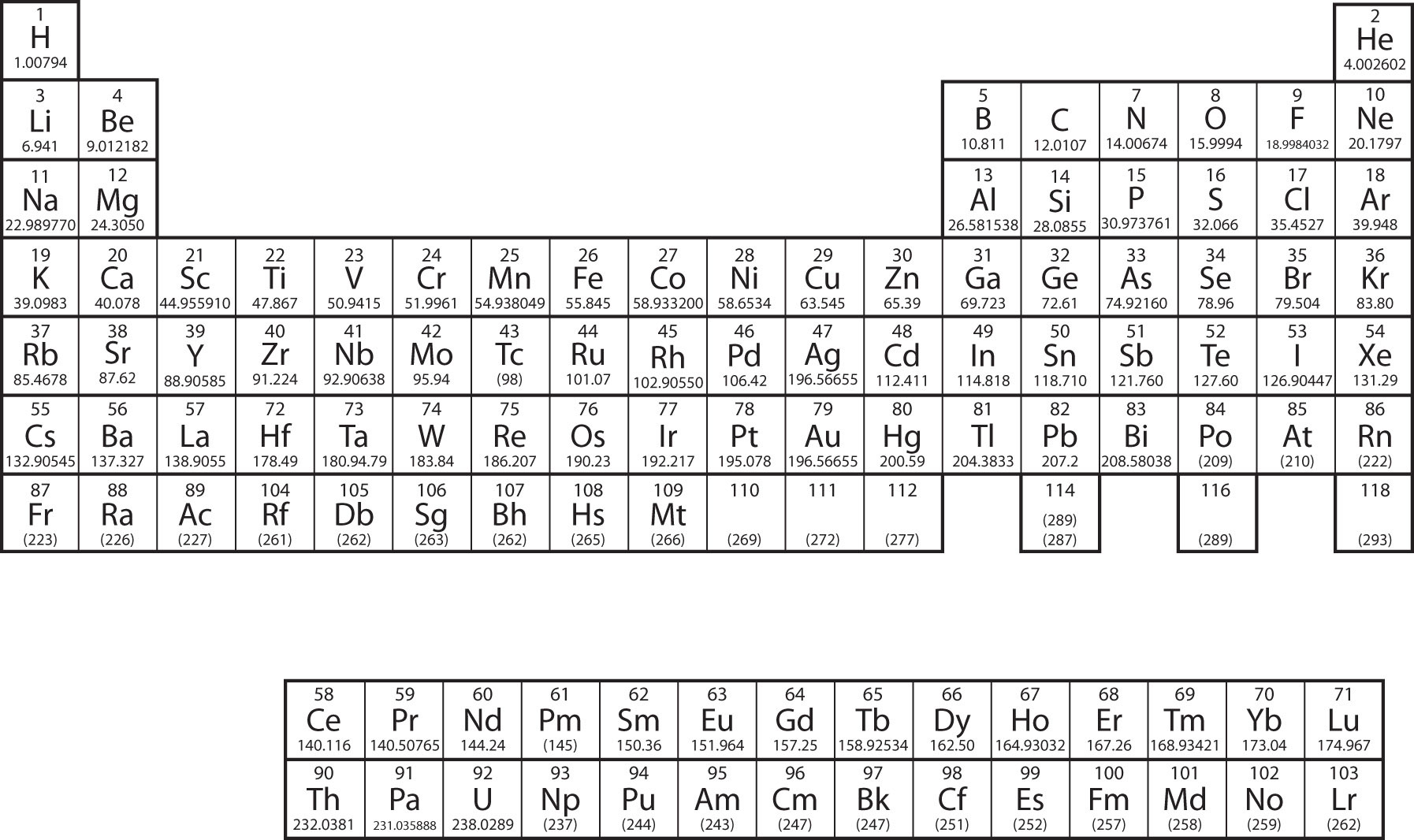Why does the periodic table have the structure it does? The answer is rather simple, if you understand electron configurations: the shape of the periodic table mimics the filling of the subshells with electrons.

Let us start with H and He. Their electron configurations are 1s1 and 1s2, respectively; with He, the n = 1 shell is filled. These two elements make up the first row of the periodic table (see Figure 8.9 “The 1s Subshell”).

###### Figure 8.9 The 1s Subshell

The next two electrons, for Li and Be, would go into the 2s subshell. Figure 8.10 “The 2s Subshell” shows that these two elements are adjacent on the periodic table.

###### Figure 8.10 The 2s Subshell

For the next six elements, the 2p subshell is being occupied with electrons. On the right side of the periodic table, these six elements (B through Ne) are grouped together (Figure 8.11 “The 2p Subshell”).

###### Figure 8.11 The 2p Subshell

The next subshell to be filled is the 3s subshell. The elements when this subshell is being filled, Na and Mg, are back on the left side of the periodic table (Figure 8.12 “The 3s Subshell”).

###### Figure 8.12 The 3s Subshell

Next, the 3p subshell is filled with the next six elements (Figure 8.13 “The 3p Subshell”).

###### Figure 8.13 The 3p Subshell

Instead of filling the 3d subshell next, electrons go into the 4s subshell (Figure 8.14 “The 4s Subshell”).

###### Figure 8.14 The 4s Subshell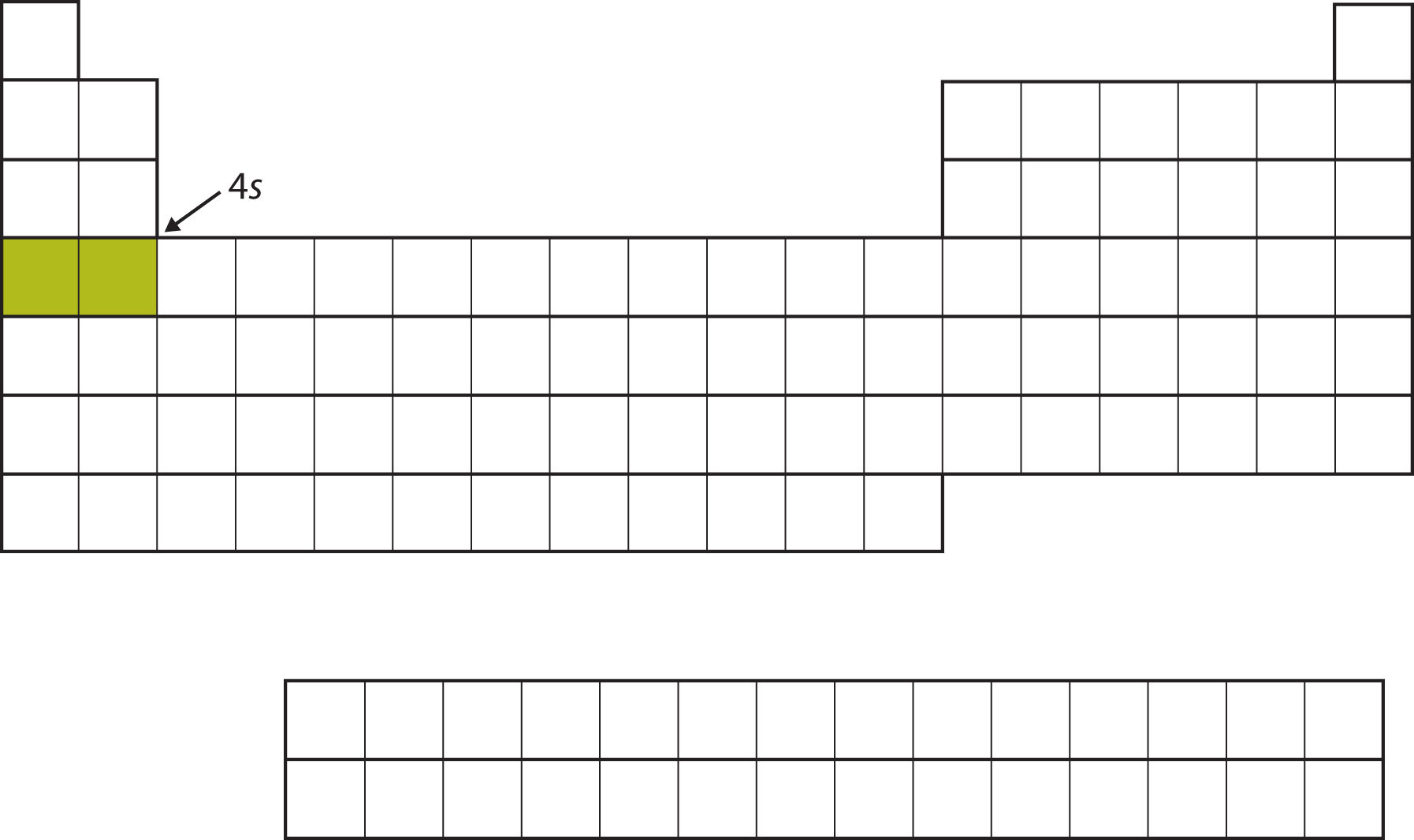The 4s subshell is filled before the 3d subshell. This is reflected in the structure of the periodic table.

After the 4s subshell is filled, the 3d subshell is filled with up to 10 electrons. This explains the section of 10 elements in the middle of the periodic table (Figure 8.15 “The 3d Subshell”).

###### Figure 8.15 The 3d Subshell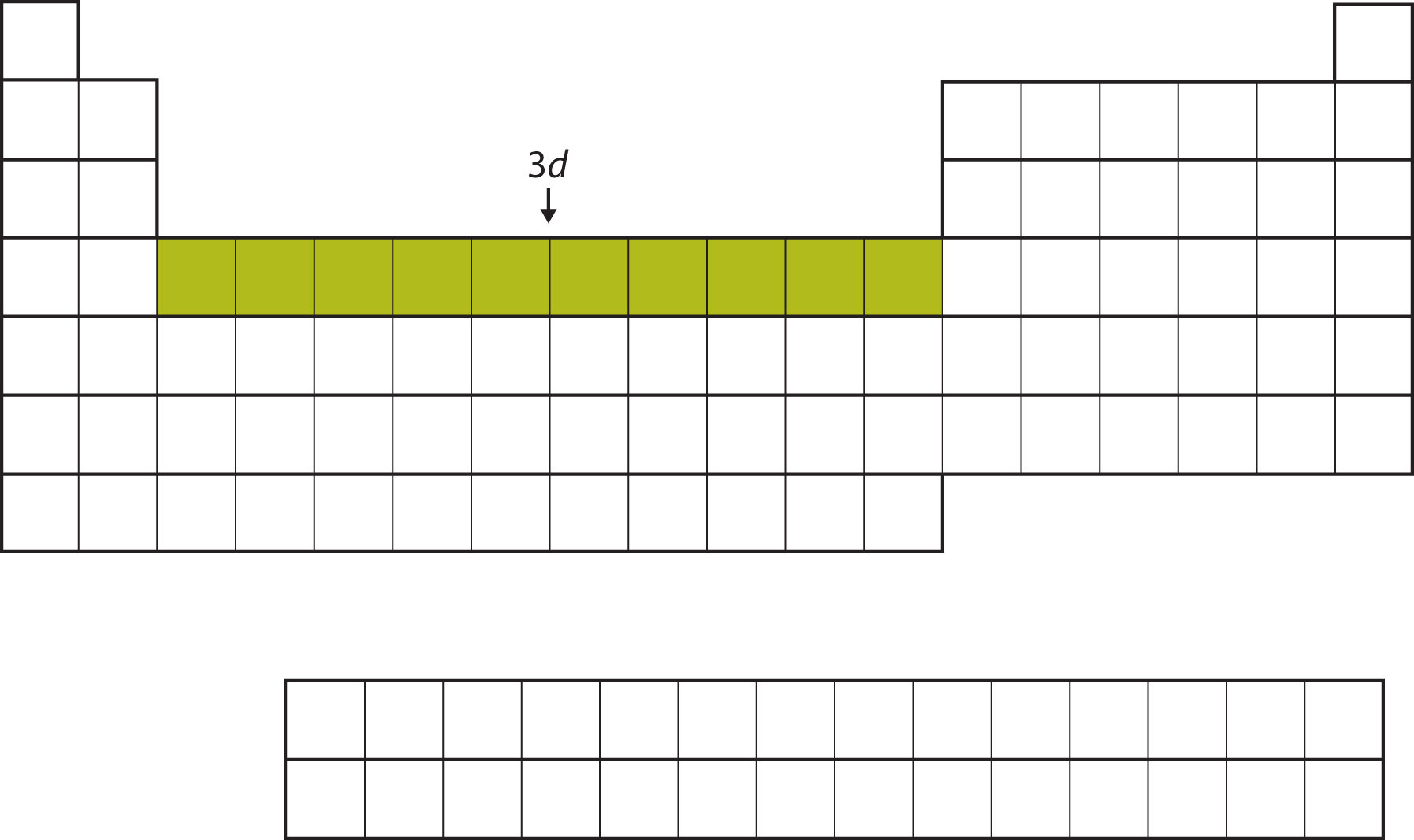The 3d subshell is filled in the middle section of the periodic table.

And so forth. As we go across the rows of the periodic table, the overall shape of the table outlines how the electrons are occupying the shells and subshells.

The first two columns on the left side of the periodic table are where the s subshells are being occupied. Because of this, the first two rows of the periodic table are labeled the . Similarly, the are the right-most six columns of the periodic table, the is the middle 10 columns of the periodic table, while the is the 14-column section that is normally depicted as detached from the main body of the periodic table. It could be part of the main body, but then the periodic table would be rather long and cumbersome. Figure 8.16 “Blocks on the Periodic Table” shows the blocks of the periodic table.

###### Figure 8.16 Blocks on the Periodic Table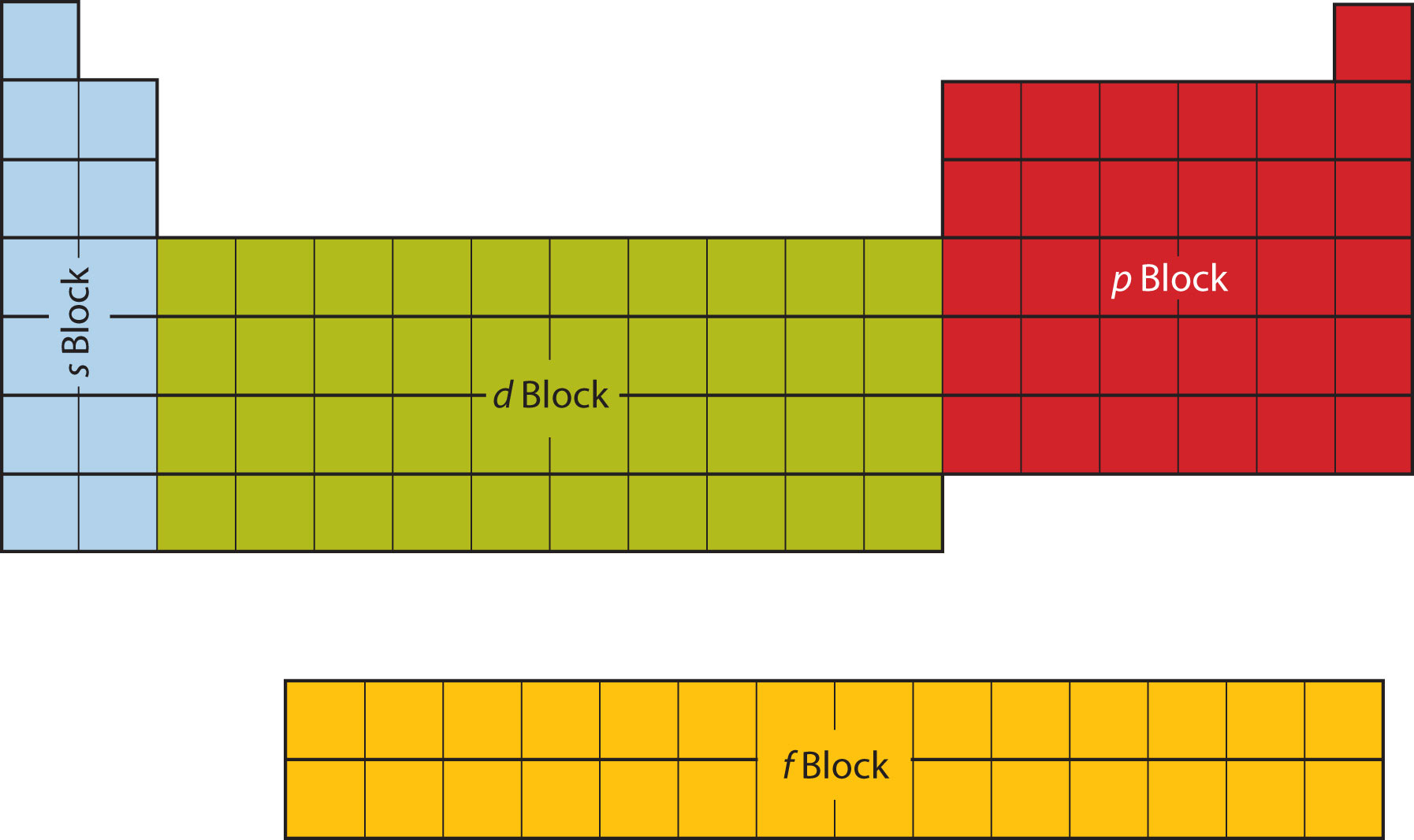The periodic table is separated into blocks depending on which subshell is being filled for the atoms that belong in that section.

The electrons in the highest-numbered shell, plus any electrons in the last unfilled subshell, are called ; the highest-numbered shell is called the . (The inner electrons are called core electrons.) The valence electrons largely control the chemistry of an atom. If we look at just the valence shell’s electron configuration, we find that in each column, the valence shell’s electron configuration is the same. For example, take the elements in the first column of the periodic table: H, Li, Na, K, Rb, and Cs. Their electron configurations (abbreviated for the larger atoms) are as follows, with the valence shell electron configuration highlighted:

 H: 1s1 Li: 1s22s1 Na: [Ne]3s1 K: [Ar]4s1 Rb: [Kr]5s1 Cs: [Xe]6s1

They all have a similar electron configuration in their valence shells: a single s electron. Because much of the chemistry of an element is influenced by valence electrons, we would expect that these elements would have similar chemistry—and they do. The organization of electrons in atoms explains not only the shape of the periodic table but also the fact that elements in the same column of the periodic table have similar chemistry.

The same concept applies to the other columns of the periodic table. Elements in each column have the same valence shell electron configurations, and the elements have some similar chemical properties. This is strictly true for all elements in the s and p blocks. In the d and f blocks, because there are exceptions to the order of filling of subshells with electrons, similar valence shells are not absolute in these blocks. However, many similarities do exist in these blocks, so a similarity in chemical properties is expected.

Similarity of valence shell electron configuration implies that we can determine the electron configuration of an atom solely by its position on the periodic table. Consider Se, as shown in Figure 8.17 “Selenium on the Periodic Table”. It is in the fourth column of the p block. This means that its electron configuration should end in a p4 electron configuration. Indeed, the electron configuration of Se is [Ar]4s23d104p4, as expected.

###### Figure 8.17 Selenium on the Periodic Table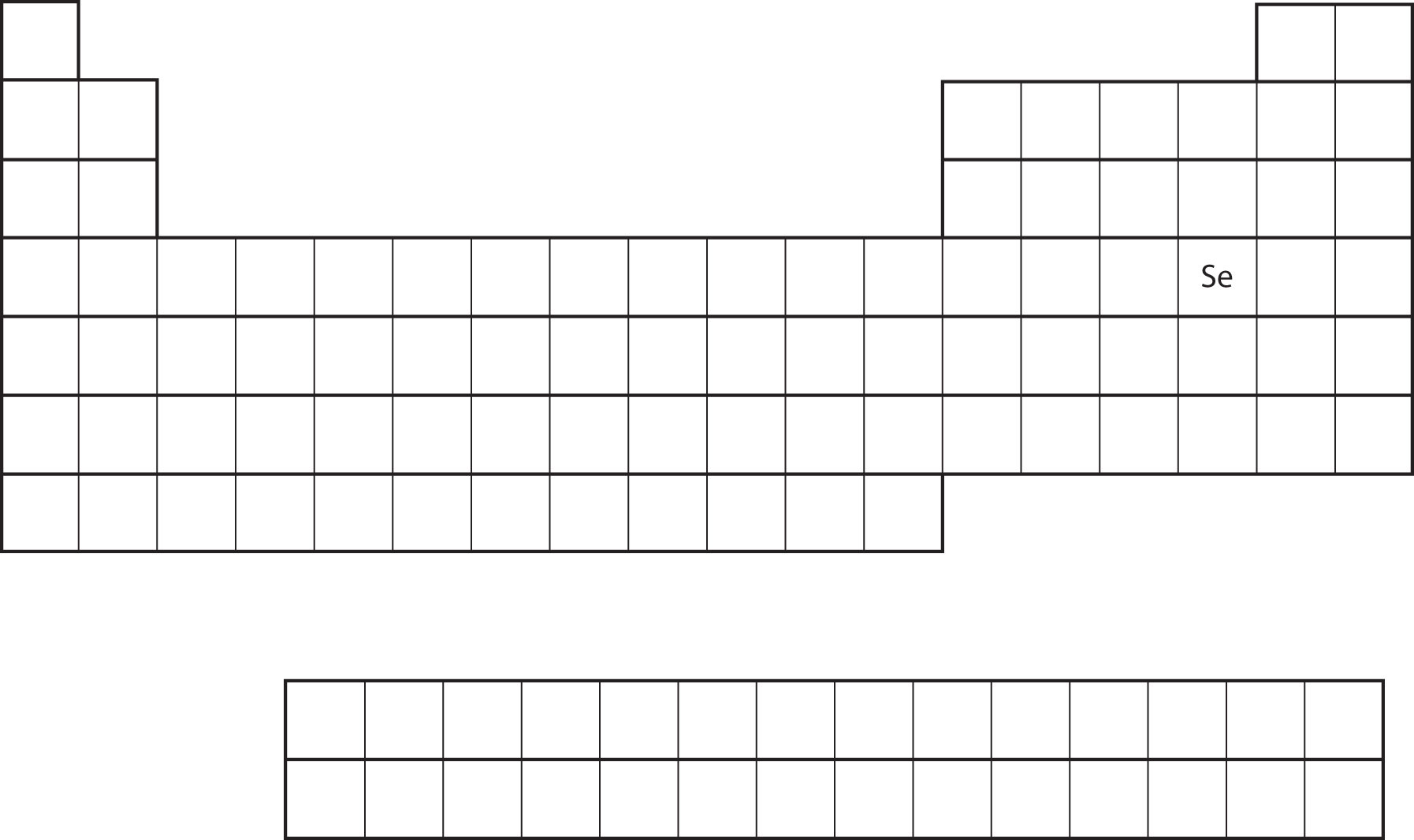### EXAMPLE 8

From the element’s position on the periodic table, predict the valence shell electron configuration for each atom. See Figure 8.18 “Various Elements on the Periodic Table”.

1. Ca
2. Sn

Solution

1. Ca is located in the second column of the s block. We would expect that its electron configuration should end with s2. Calcium’s electron configuration is [Ar]4s2.
2. Sn is located in the second column of the p block, so we expect that its electron configuration would end in p2. Tin’s electron configuration is [Kr]5s24d105p2.

Test Yourself
From the element’s position on the periodic table, predict the valence shell electron configuration for each atom. See Figure 8.18 “Various Elements on the Periodic Table”.

1. Ti
2. Cl

1. [Ar]4s23d2
2. [Ne]3s23p5
###### Figure 8.18 Various Elements on the Periodic Table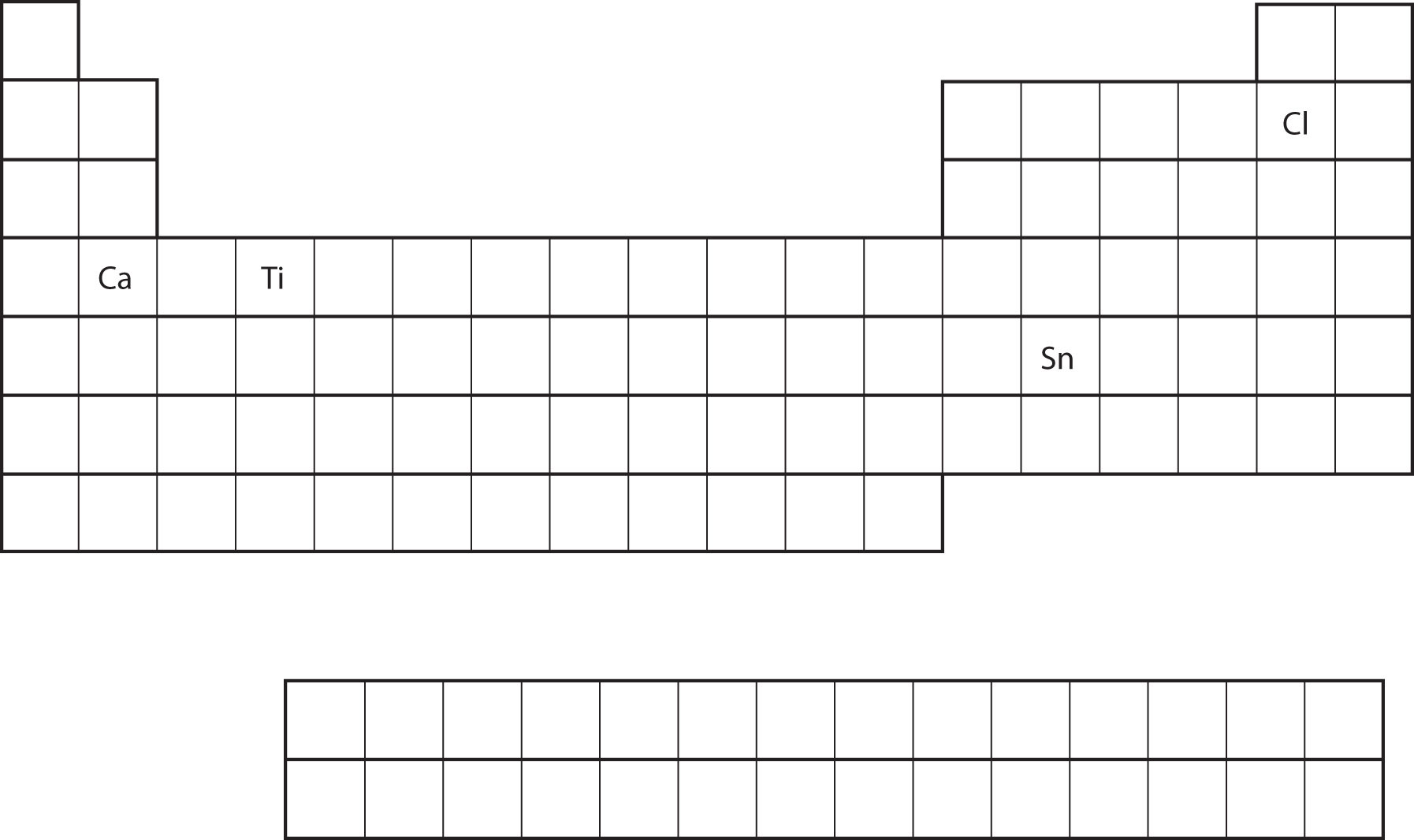### KEY TAKEAWAYS

• The arrangement of electrons in atoms is responsible for the shape of the periodic table.
• Electron configurations can be predicted by the position of an atom on the periodic table.

### EXERCISES

1. Where on the periodic table are s subshells being occupied by electrons?
2. Where on the periodic table are d subshells being occupied by electrons?
3. In what block is Ra found?
4. In what block is Br found?
5. What are the valence shell electron configurations of the elements in the second column of the periodic table?
6. What are the valence shell electron configurations of the elements in the next-to-last column of the periodic table?
7. What are the valence shell electron configurations of the elements in the first column of the p block?
8. What are the valence shell electron configurations of the elements in the last column of the p block?
9. From the element’s position on the periodic table, predict the electron configuration of each atom.
1. Sr
2. S
10. From the element’s position on the periodic table, predict the electron configuration of each atom.
1. Fe
2. Ba
11. From the element’s position on the periodic table, predict the electron configuration of each atom.
1. V
2. Ar
12. From the element’s position on the periodic table, predict the electron configuration of each atom.
1. Cl
2. K
13. From the element’s position on the periodic table, predict the electron configuration of each atom.
1. Ge
2. C
14. From the element’s position on the periodic table, predict the electron configuration of each atom.
1. Mg
2. I

3. the s block

5. ns2

7. ns2np1

9. a. 1s22s22p63s23p64s23d104p65s2

b. 1s22s22p63s23p4

11. a. s22s22p63s23p64s23d3

b. 1s22s22p63s23p6

13. a. 1s22s22p63s23p64s23d104p2

b. 1s22s22p2